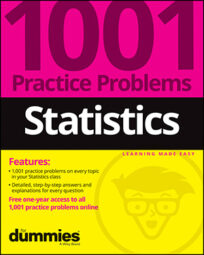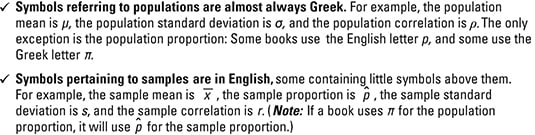##### Statistics: 1001 Practice Problems For Dummies (+ Free Online Practice)There are many types of statistics problems, including the use of pie charts, bar graphs, means, standard deviation to correlation, regression, confidence intervals, and hypothesis tests.

To be successful, you need to be able to make connections between statistical ideas and statistical formulas. Through practice, you see what type of technique is required for a problem and why, as well as how to set up the problem, work it out, and make proper conclusions.

Most statistics problems you encounter likely involve terminology, symbols, and formulas. No worries! This Cheat Sheet gives you tips for success.

## Terminology used in statistics

Like every subject, statistics has its own language. The language is what helps you know what a problem is asking for, what results are needed, and how to describe and evaluate the results in a statistically correct manner. Here’s an overview of the types of statistical terminology:

• Four big terms in statistics are population, sample, parameter, and statistic:

• A population is the entire group of individuals you want to study, and a sample is a subset of that group.

• A parameter is a quantitative characteristic of the population that you’re interested in estimating or testing (such as a population mean or proportion).

• A statistic is a quantitative characteristic of a sample that often helps estimate or test the population parameter (such as a sample mean or proportion).

• Descriptive statistics are single results you get when you analyze a set of data — for example, the sample mean, median, standard deviation, correlation, regression line, margin of error, and test statistic.

• Statistical inference refers to using your data (and its descriptive statistics) to make conclusions about the population. Major types of inference include regression, confidence intervals, and hypothesis tests.

## Breaking down statistical formulas

Formulas abound in statistics problems — there’s just no getting around them. However, there’s typically a method to the madness if you can break the formulas into pieces. Here are some helpful tips:

• Formulas for descriptive statistics basically take the values in the data set and apply arithmetic operations. Often, the formulas look worse than the process itself. The key: If you can explain to your friend how to calculate a standard deviation, for example, the formula is more of an afterthought.

• Formulas for the regression line have a basis in algebra. Instead of the typical y = mx + b format everyone learns in school, statisticians use y = a + bx.

• The slope, b, is the coefficient of the x variable.

• The y-intercept, a, is where the regression line crosses the y-axis.

The formulas for finding a and b involve five statistics: the mean of the x-values, the mean of the y-values, the standard deviations for the x‘s, the standard deviations for the y‘s, and the correlation.

• All the various confidence interval formulas, when made into a list, can look like a hodge-podge of notation. However, they all have the same structure: a descriptive statistic (from your sample) plus or minus a margin of error. The margin of error involves a z*-value (from the Z-distribution) or t*-value (from the t-distribution) times the standard error. The parts you need for standard error are generally provided in the problem, and the z*- or t*-values come from tables.

• Hypothesis tests also have a common structure. Although each one involves a series of steps to carry out, they all boil down to one thing: the test statistic. A test statistic measures how far your data is from what the population supposedly looks like. It takes the difference between your sample statistic and the (claimed) population parameter and standardizes it so you can look it up on a common table and make a decision.

## Symbols used in statistics

Symbols (or notation) found in statistics problems fall into three categories: math symbols, symbols referring to a population, and symbols referring to a sample. Math symbols are easy enough to decipher with a simple review of algebra; they involve items such as square root signs, equations of a line, and combinations of math operations. The other two categories are a bit more challenging, and knowing the difference between them is critical.## Stick to a strategy when you solve statistics problems

Solving statistics problems is always about having a strategy. You can’t just read a problem over and over and expect to come up with an answer — all you’ll get is anxiety! Although not all strategies work for everyone, here’s a three-step strategy that has proven its worth:

1. Label everything the problem gives you.

For example, if the problem says “X has a normal distribution with a mean of 10 and a standard deviation of 2,” leap into action: Circle the 10 and write μ, and circle the 2 and write σ. That way you don’t have to hunt later to find the numbers you need.

2. Write down what you’re asked to find in a statistical manner.

Hint: Questions typically tell you what they want in the last line of the problem. For example, if you’re asked to find the probability that more than 10 people come to the party, write “Find P(X > 10).”

3. Use a formula, a process, or an example you’ve seen to connect what you’re asked to find with what the problem gives you.

For example, suppose you’re told that X has a normal distribution with a mean of 80 and a standard deviation of 5, and you want the probability that X is less than 90. Label what you’re given: “X normal with μ = 80 and σ = 5.” Next, write what you need to find, using symbols: “Find P(X < 90).” Because X has a normal distribution and you want a probability, the connection is the Z-formula: Z = (Xμ)/σ. You have a good idea that this is the right formula because it includes everything you have: μ, σ, and the value of X (which is 90). Find P(X < 90) = P[Z < (90 – 80)/5] = P(Z < 2) = 0.9772. Voilà!